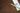•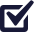•Divide into areas
•Measure
•Do the math

Learn the basics of measuring your site with these four easy steps.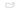#### Step 1: Sketch your site

Using pen and paper, replicate your site as a basic line drawing. It only needs to be a concept drawing for you to note the measurements you’re about to take.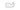#### Step 2: Divide into areas

If your site features multiple sections, bends and / or curves, divide them into suitable sections as rectangles.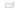#### Step 3: Measure

Using a tape measure, record the actual length and width of each section, as indicated on your concept drawing.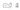#### Step 4: Do the math

Using the length and width, calculate the square meterage of each section by multiplying them together. Now simply add the sum of all sections together to discover your total area in m2.

## Neead a help for you lawn project?

Twin View Turf Pty Ltd © 2023